# 16+ Unit Circle Chart Templates

Everybody knows about the history of the wheel. The revolutionary invention influenced the world more than any other thing. Unit Circle Templates are nonetheless important in mathematics. Circles with a unit radius or the radius of 1 are called Unit circles. Are you in need of unit circles? Stop reinventing the wheel. Rather, try to search for some sample Unit Wheel Templates online. Here you can download various copies of Unit Circles that help you with multiple mathematical functions.

## 8 Unit Circle Chart Templates Bundle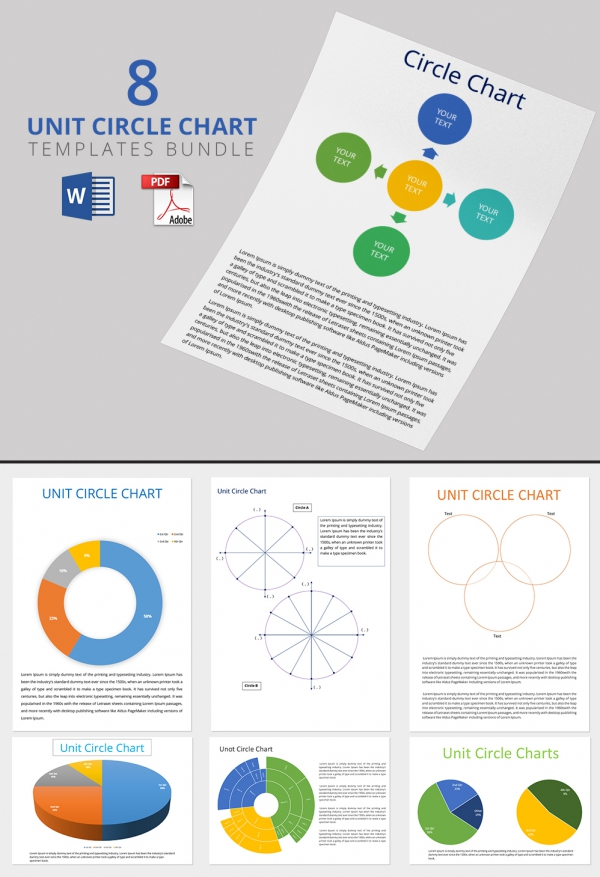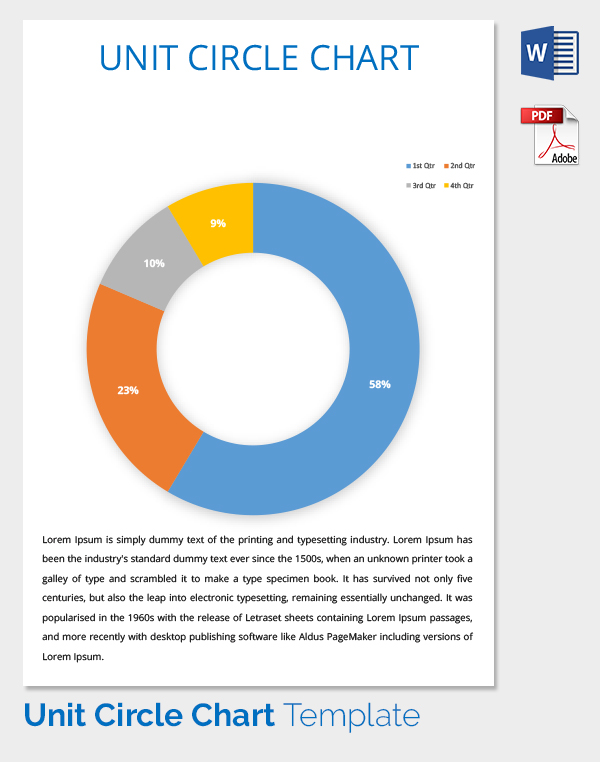## Simple Unit Circle Chart Template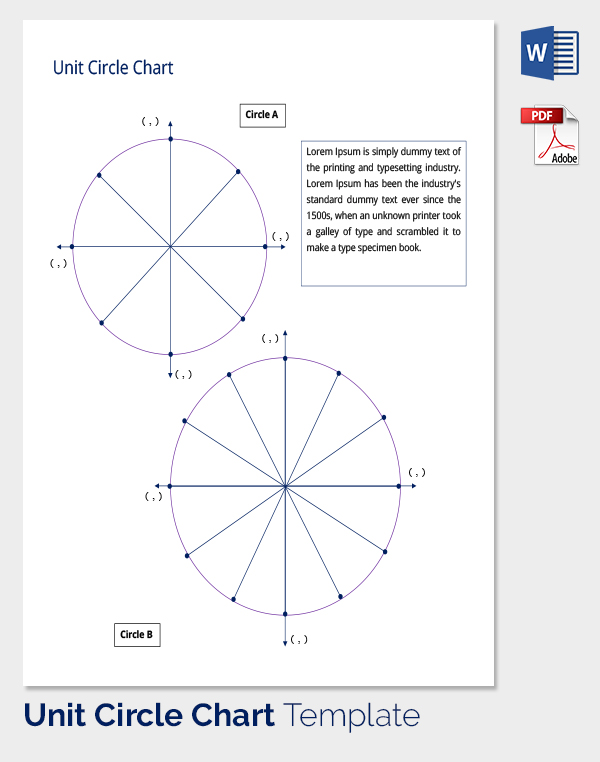## Editable Unit Circle Chart Template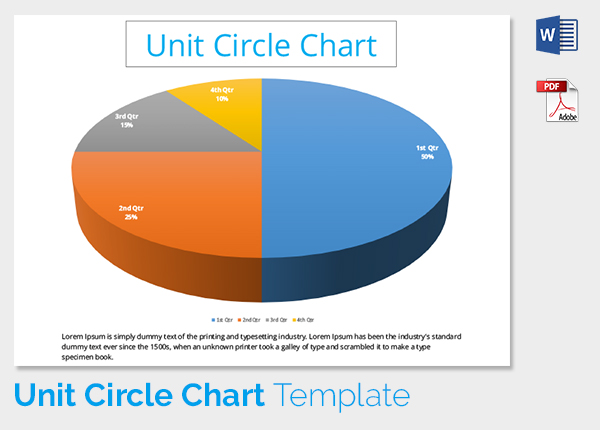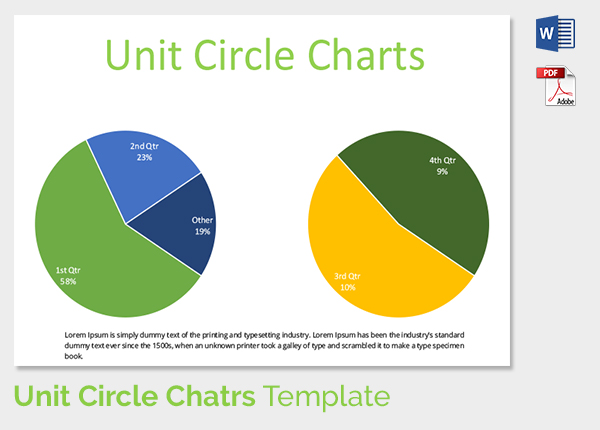## Printable Unit Circle Chart Template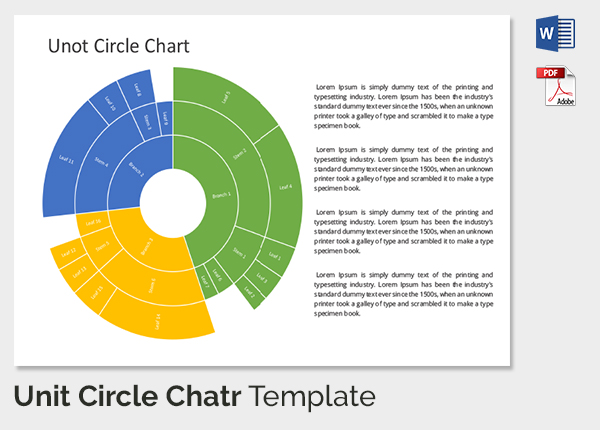## Editable Unit Circle Chart Template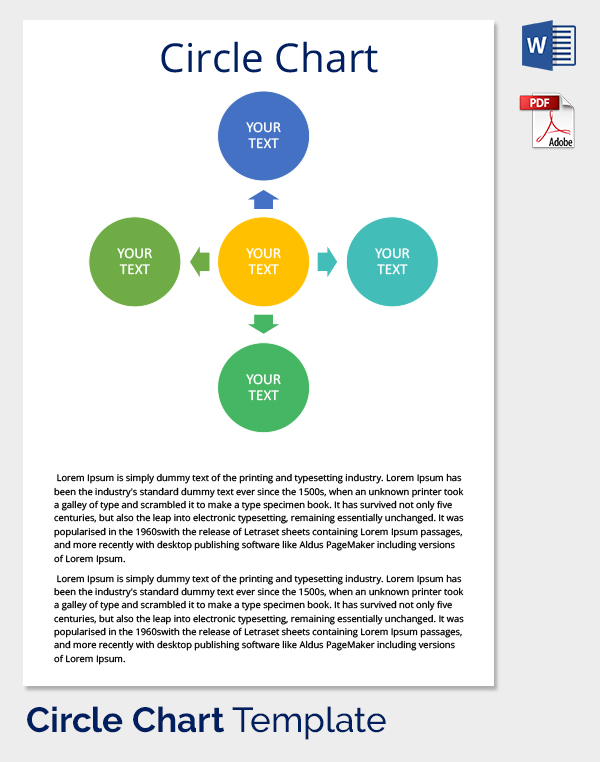## Unit Circle Chart Template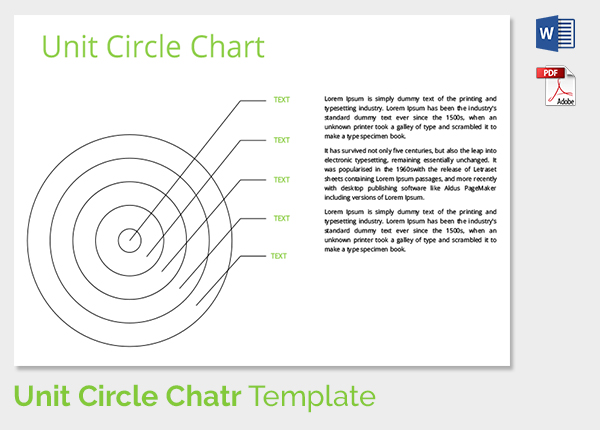## Blank Unit Circle Chart Template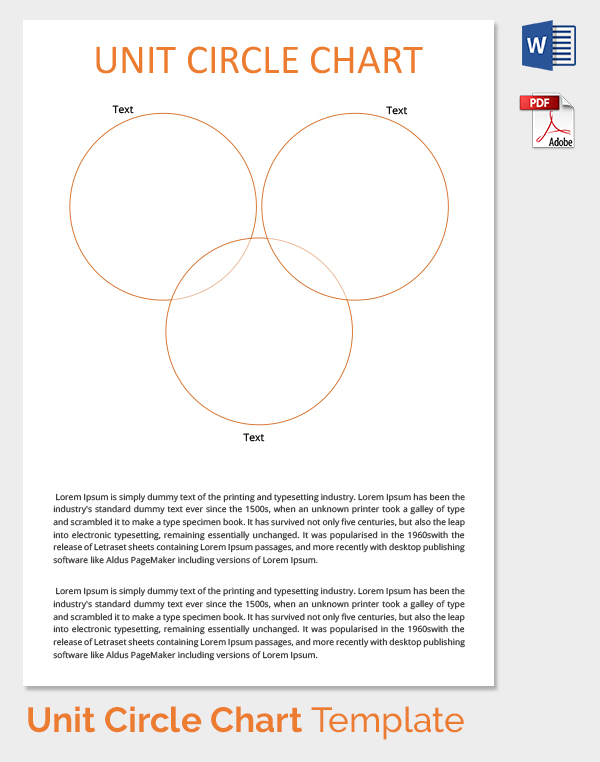## Trig Unit Circle Chart Template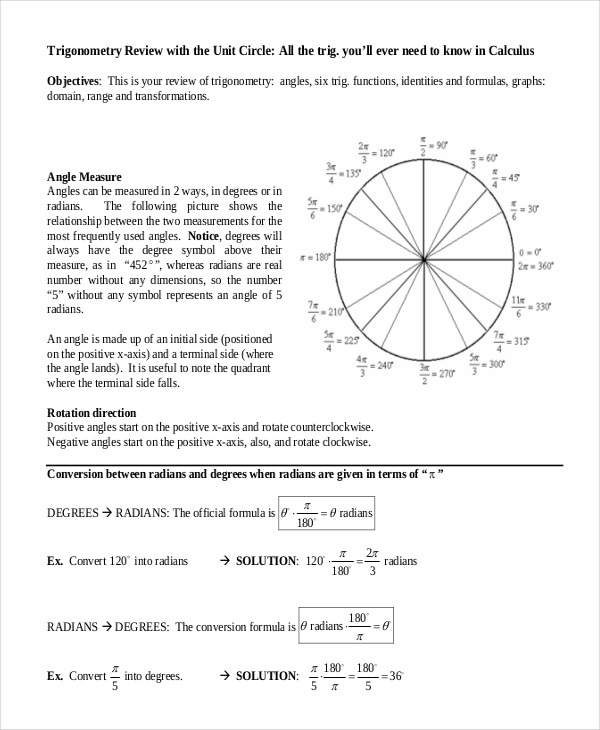pstcc.edu

In a circle, a radian is defined by the radius of a circle. All the trigonometric functions and equations depend on it and therefore many choose using Unit Circle for the trigonometric calculations. Our Trig Unit Circle Chart Templates discuss the arc length and other trigonometric ratios. They ensure that the teaching and learning of trigonometry are delivered with ease. You may also see Venn Diagram Template.

## Interactive Unit Circle Template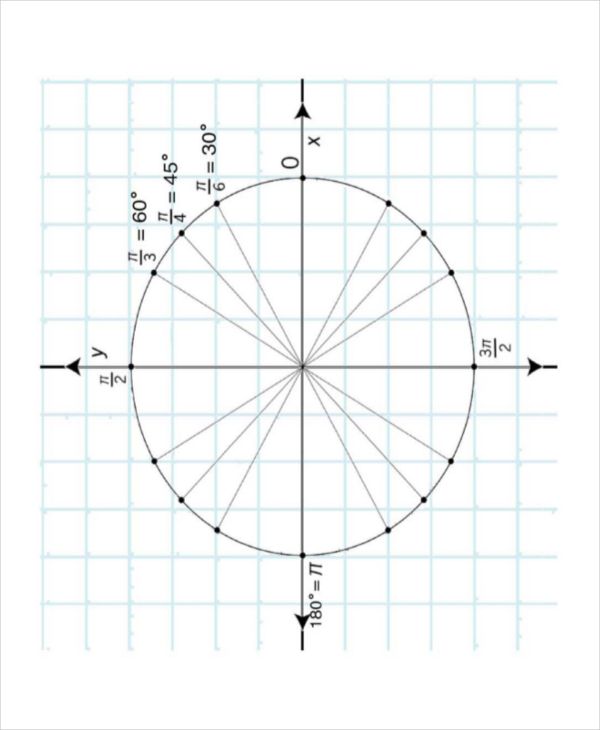ocw.mit.edu

How good are you with trigonometric equations? If you are that chap who is not so dexterous with the equations, then you can use triangles and/or tables. But if you are really smart enough, you should opt for Interactive Unit Circle Templates. They talk about the domain and the period of Sine and Cosine.

## Complete Unit Circle Template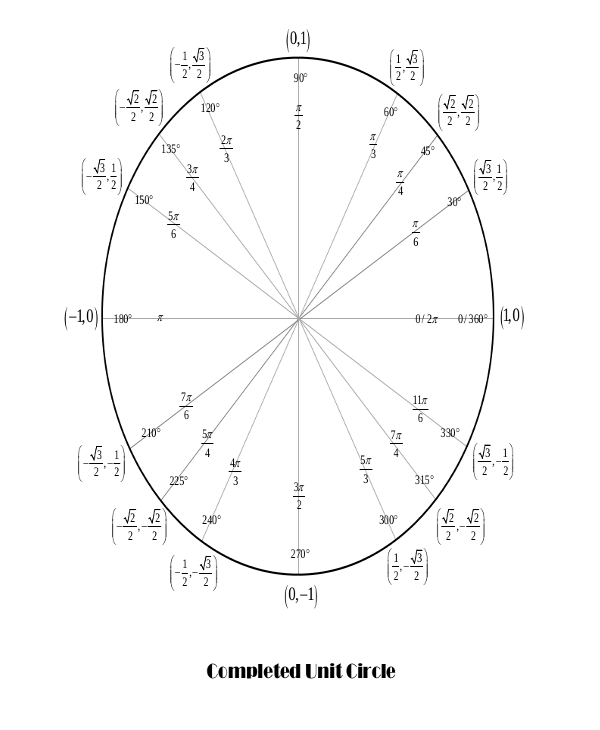gradeamathhelp.com

Are you thinking of throwing a Unit Circle to your students as an assignment? Then use these Complete Unit Circle Templates. Download it and get it printed for the whole classroom. Ask your students to practice it until they are able to finish it in 5 minutes.

## Tan Unit Circle Template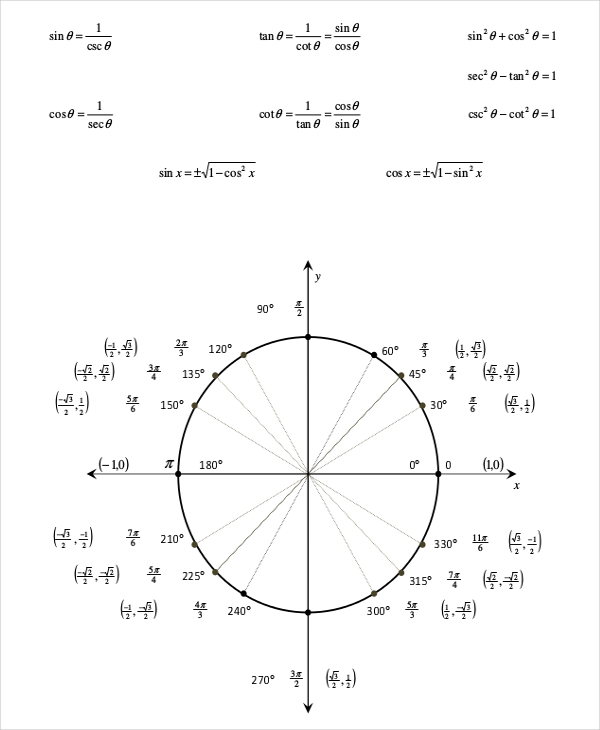mcckedu

Tan Unit Circle Templates help you solve tangent of angles 300, 450, and 600. For example, Sin 30 = 1/2, cos 30 = /2, thus Tan 30 = 1/, Tan 45 = 1 and tan 60 = ,while cos 90 =0 and sin 90 =1. Tan 90 is unknown.

## Full Unit Circle Template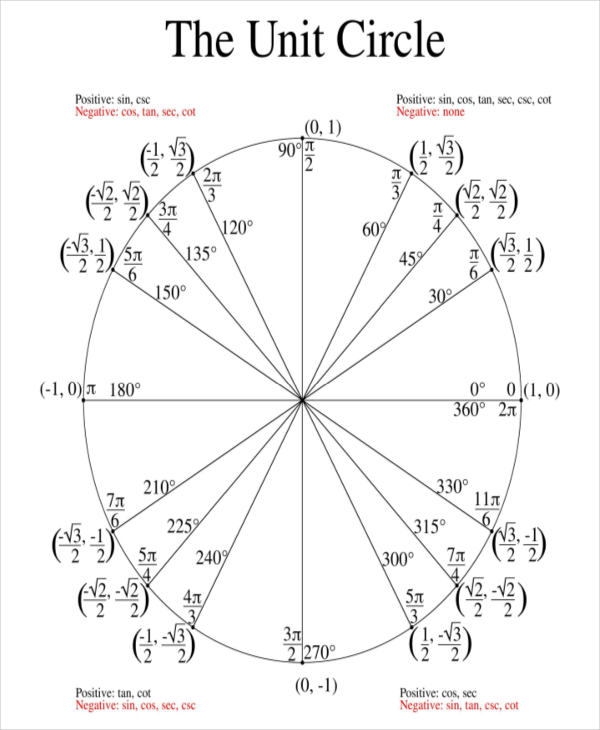collinshill.org

What’s the value of cos 3/2? If you have no idea, get an idea in the form of these full unit circle templates. They are designed to do wonders in the trigonometry class without getting stressed. They reduce the stress of educators and make learning more interesting and playful. These circle templates are thoughtfully yours.

## Empty Unit Circle Template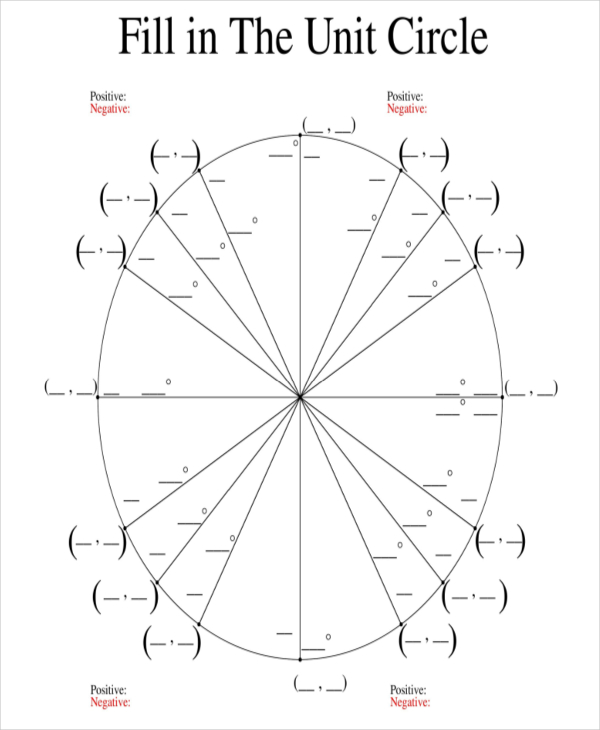math.utah.edu

Empty Unit Circles are often called as Practice filling Unit Circles. These are best used for practicing equations and fractions. Each gray line in our Empty Unit Circle Templates resembles a mostly used angle in Trigonometry. Have an interactive quiz on degrees, radians, unit circles, etc. The more you practice the circle, the better you will be at Trigonometry.

## Printable Unit Circle Template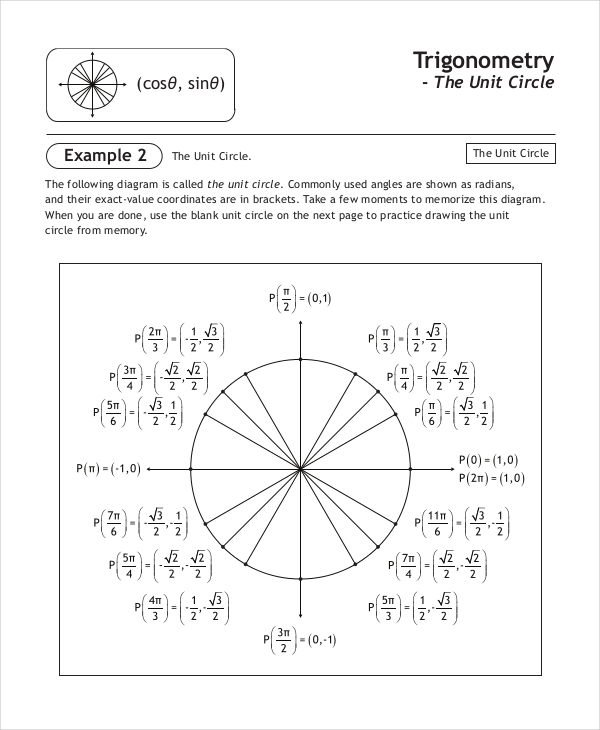math30.ca

Printable Unit Circles are basic Unit Circles. They allow you to design the circles right from the beginning. Our Printable Unit Circle Templates are print-ready. They can be your best classroom practices. They are available in multiple designs and formats.You may also see Venn Diagram Templates.

### > What is the Terminal Side of the Unit Circle?

We all know that it needs two rays to form an angle. In trigonometry, the coordinating ray that is always placed on the y-axis is called terminal side of the angle. The way the angle is positioned is called standard position. The terminal side will intersect the circle at one or the other point. You may also see Ecomap Template.

### > What is an Open Unit Disk and a Closed Unit Disk?

The interior of the unit circle is called the open unit disk, while the interior of the unit circle combined with the unit circle itself is called the closed unit disk. In mathematics, the open unit disk around p is the set of points whose distance from p is less than 1. The closed unit disk around p is the set of points whose distance from p is less than or equal to 1.